# KSEEB Solutions for Class 7 Maths Chapter 4 Simple Equations Ex 4.2

Students can Download Class 7 Maths Chapter 4 Simple Equations Ex 4.2  Questions and Answers, Notes Pdf, KSEEB Solutions for Class 7 Maths helps you to revise the complete Karnataka State Board Syllabus and to clear all their doubts, score well in final exams.

## Karnataka State Syllabus Class 7 Maths Chapter 4 Simple Equations Ex 4.2

Question 1.
Given first the step you will use to separate the variable and then solve the equation:
a) x – 1 = 0
Solution:
The given equation = x – 1 = 0
Add 1 to both the sides
x – 1 + 1 = 0 + 1 ⇒ x = 1
Checking
x – 1 = 0
put the value of x = 1
1 – 1 = 0
∴ LHS = RHS (checked)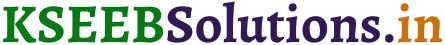b) x + 1 = 0
The given equation = x + 1 = 0
Subtract 1 from both the sides
x – 1 + 1  = 0 – 1 ⇒ x = – 1
Verification
x+ 1 = 0
substitute the value of x = – 1 then x + 1 = 0
(-1) + 1 = 0
0 = 0
LHS = RHS (verified)

c) x – 1 = 5
The given equation is x – 1 = 5
x – 1 + 1 = 5 + 1 ⇒ x = 6
Substitute the value in the equation x = 6.
x – 1 = 5
6 – 1 = 5
5 = 5
∴ LHS = RHS (verified)

d) x + 6 = 2
The given equation is x + 6 = 2
Subtract 6 from both the sides
x  + 6 – 6 = 2 – 6 ⇒ x = – 4
Substitute the value of x = – 4 in the given equation,
x + 6 = 2
– 4 + 6 = 2
2 = 2
∴ LHS = RHS (verified)

e) y – 4 = -7
The given equation is y –  4 = – 7
Add 4 to both the sides
y – 4 + 4 = – 7 + 4 ⇒ y = – 3
substitute the value of y = – 3 in the given equation
y – 4 = -7
– 3 – 4 = – 7
– 7 = – 7
∴ LHS = RHS (verified)

f) y – 4 = 4
Solution:
The given equation is y – 4 = 4
Add 4 to both the sides
y – 4 + 4 = 4 + 4  ⇒ y = 8
substitute the value of y = 8 in the given equation
y –  4 = 4
8 – 4 = 4
4 = 4
∴ LHS = RHS (verified)g) y + 4 = 4
Solution:
The given equation is y + 4 = 4
subtract 4 from both sides
= y – 4 + 4 = 4 – 4 ⇒ y = 0
substitute the value of y = 0 in the given equation.
y + 4 = 4
0 + 4 = 4
4 = 4
∴ LHS = RHS (verified)

h) y + 4 = – 4
Solution:
The given equation is y + 4 = – 4
subtract 4 from both the sides
y – 4 + 4 = – 4 – 4
y = – 8 (verified)
substitute the value of y = – 8 in the given equation
y + 4 = – 4
– 8 + 4 = – 4
– 4 = – 4
∴ LHS = RHS (verified)

Question 2.
Give first the step you will use to separate the variable and then solve the equation:

a) 3l = 42
Solution:
The given equation is 3l = 42
Divide by 3 both the sides
$$\frac{31}{3}$$ = $$\frac{42}{3}$$
∴ l = 14
Substitute the value of l = 14 in the given equation
3l = 42
3(14) = 42
42 = 42
∴ LHS = RHS (verified)

b) $$\frac{\mathbf{b}}{2}$$ = 6
Solution:
The given equation is $$\frac{\mathbf{b}}{2}$$ = 6
Multiplied by 2 both the sides b
$$\frac{b}{2}$$ × 2 = 6 × 2
∴ b = 12
substitute the value of b = 12 in the given equation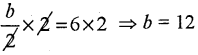∴ LHS = RHS (verified)

c) $$\frac{\mathbf{p}}{7}$$ = 4
Solution:
The given equation is $$\frac{p}{7}$$ = 4
Multiplied by 7 to both the sides
$$\frac{p}{7}$$ ×7 = 4 × 7
∴ p = 28
Substitute the value of p = 28 in the given equation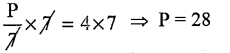∴ LHS = RHS (verified)

d) 4x = 25
Solution:
The given equation is 4 x = 25
Divide both sides by 4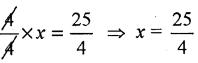substitue the value of x = $$\frac{25}{4}$$ in the given equation
4x = 25
4 × $$\frac{25}{4}$$ = 25
∴ LHS = RHS (verified)

e) 8y = 36
Solution:
The given equation is 8y = 36
Divide both sides by 8Substitute the equation by y = $$\frac{9}{2}$$ in the given equation
8y = 36∴ LHS = RHSf) $$\frac{z}{3}=\frac{5}{4}$$
Solution:
Multiply both sides by 3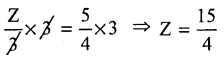g) $$\frac{a}{5}=\frac{7}{15}$$
Solution:
Multiply both sides by 5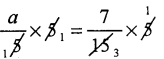Substitute the value of a = $$\frac{7}{3}$$ in the given equation

h) 20t = – 10
Solution:
The given equation is 20 t = – 10
Divide by 20 to both sidesQuestion 3.
Given the steps you will use to separate the variable and then solve the equation:
a) 3n – 2 = 46
Solution:
Add 2 to both sides 3n – 2 + 2 = 46 + 2
3n = 48
Divide the equation by 3
$$\frac{3n}{3}$$ = $$\frac{48}{3}$$
∴ n = 16 This is required solution.
substitute the value of n = 16 in the given equation
3n – 2 = 46
3(16) – 2 = 46
48 – 2 = 46
46 = 46
∴ LHS = RHS (verified)

b) 5m + 7 = 17
The given equation is 5m + 7 = 17
Subtract 7 from both sides
5m + 7 – 7 = 17 – 7
5m = 10
Divide the equation by 5 both the sides
$$\frac{5m}{5}$$ = $$\frac{10}{5}$$
∴ m = 2 (solution)
Substitute the value of m = 2 in the given equation
5m + 7 = 17
5(2) + 7 =17
10 + 7 = 17
∴ LHS = RHS (verified)c) $$\frac{20 p}{3}$$ = 40
Solution:
Multiply both sides by 3
$$\frac{20}{3}$$ × 3 × P = 40 × 3
20P = 120
Divide both sides by 20
$$\frac{20}{20}$$ × P = $$\frac{120}{20}$$ ⇒ P = 6

d) $$\frac{3 P}{10}=6$$
Solution:
Multiply by 10 to both the sidesDivide by 3 to both sides
$$\frac{3}{3}$$ × P = $$\frac{60}{3}$$ ⇒ P = 20

Question 4.
Solve the following equations :
a) 10p = 100
Solution: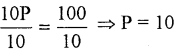b) 10p + 10 = 100
Solution:
10 P + 10 – 10  = 100 – 10
10 P = 90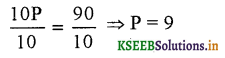c) $$\frac{\mathbf{p}}{4}$$ = 5
Solution:
$$\frac{p}{4}$$ × 4 = 5 × 4 ⇒ P = 20

d) $$\frac{-p}{3}$$ = 5
Solution:
– P = 15 ⇒ P = – 15

e) $$\frac{3 P}{4}=6$$
Solution: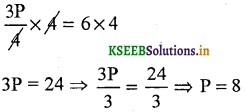f) 3s = – 9
Solution:
$$\frac{3s}{3}=\frac{-9}{3}$$
S = – 3

g) 3s + 12 = 0
Solution:
3S + 12 + 12 – 12 = 0 – 12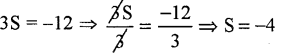h) 3s = 0
Solution: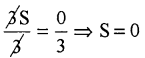j) 2q – 6 = 0
Solution:
2q – 6 + 6 = 0 + 6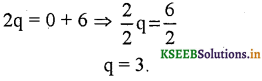k) 2q + 6 = 0
Solution:
2q + 6 + 6 = 0 – 6
2q = – 6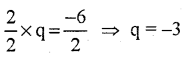l) 2q + 6 = 12
2q + 6 – 6 = 12 – 6
2q = 6